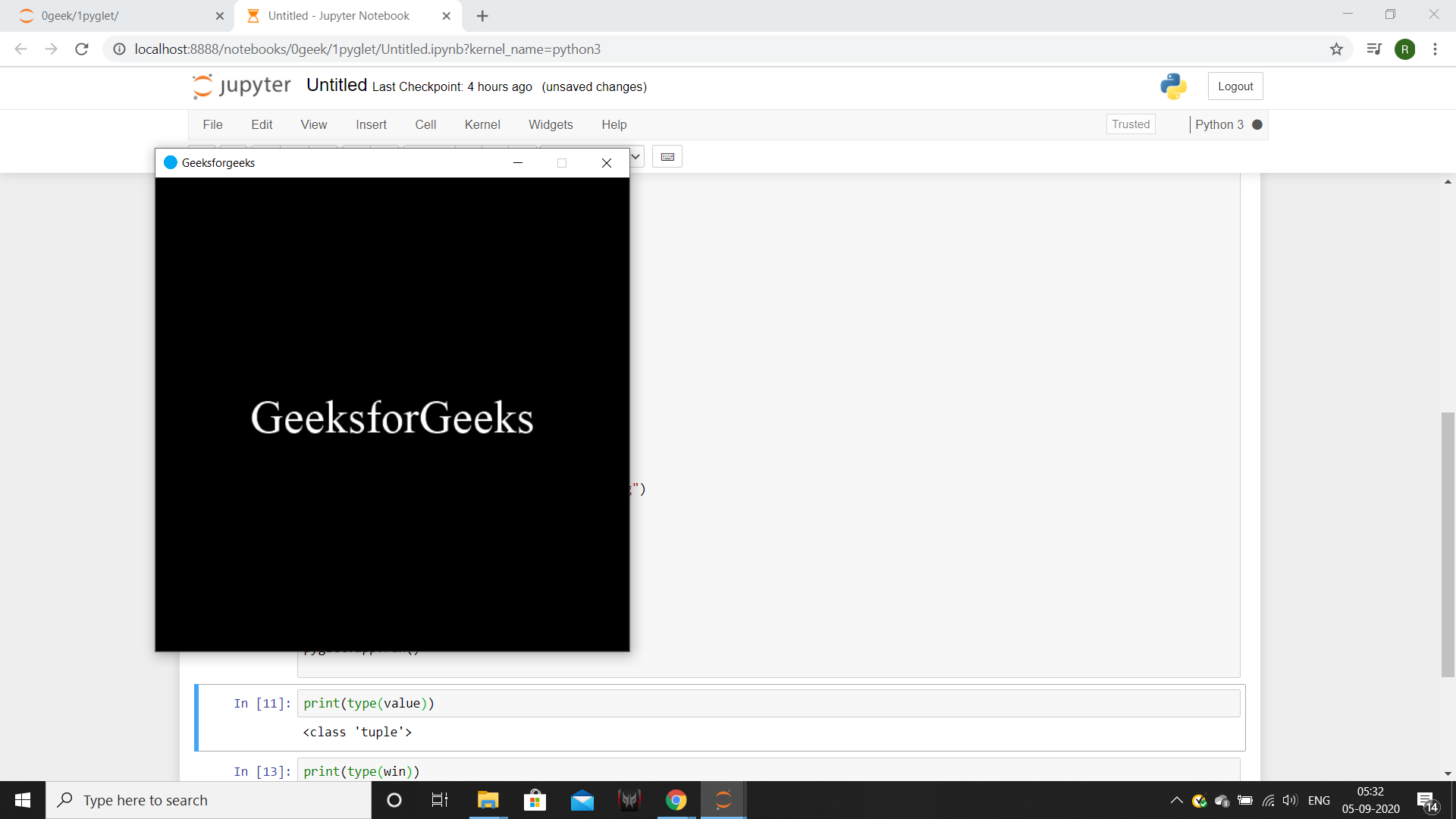# PYGLET – Setting Minimum Window Size

• Last Updated : 28 Jan, 2022

In this article we will see how we can set the minimum window size in PYGLET module in python. Pyglet is easy to use but powerful library for developing visually rich GUI applications like games, multimedia etc. A window is a “heavyweight” object occupying operating system resources. Windows may appear as floating regions or can be set to fill an entire screen (fullscreen). Once minimum size is set, the user will not be able to resize the window smaller than the given dimensions. There is no way to remove the minimum size constraint on a window (but you could set it to 0, 0). The behaviour is undefined if the minimum size is set larger than the current size of the window.
We can create a window with the help of command given below

` pyglet.window.Window(width, height, title)`

In order to create window we use set_minimum_size method with the window object
Syntax : window.set_minimum_size(width, height)
Argument : It takes two integer as argument
Return : It returns None

Below is the implementation

## Python3

 `# importing pyglet module``import` `pyglet``import` `pyglet.window.key` `# width of window``width ``=` `500` `# height of window``height ``=` `500` `# caption i.e title of the window``title ``=` `"Geeksforgeeks"` `# creating a window``window ``=` `pyglet.window.Window(width, height, title)` `# text``text ``=` `"GeeksforGeeks"` `# creating a label with font = times roman``# font size = 36``# aligning it to the center``label ``=` `pyglet.text.Label(text,``                          ``font_name ``=``'Times New Roman'``,``                          ``font_size ``=` `36``,``                          ``x ``=` `window.width``/``/``2``, y ``=` `window.height``/``/``2``,``                          ``anchor_x ``=``'center'``, anchor_y ``=``'center'``)` `# on draw event``@window``.event``def` `on_draw():``    ` `    ``# clearing the window``    ``window.clear()``    ` `    ``# drawing the label on the window``    ``label.draw()``    ` `# key press event   ``@window``.event``def` `on_key_press(symbol, modifier):``    ` `    ``# key "C" get press``    ``if` `symbol ``=``=` `pyglet.window.key.C:``        ` `        ``# close the window``        ``window.close()` `# image for icon``img ``=` `image ``=` `pyglet.resource.image("logo.png")` `# setting image as icon``window.set_icon(img)` `# setting minimum size of the window``window.set_minimum_size(``500``, ``500``)` `               ` `# start running the application``pyglet.app.run()`

Output :My Personal Notes arrow_drop_up